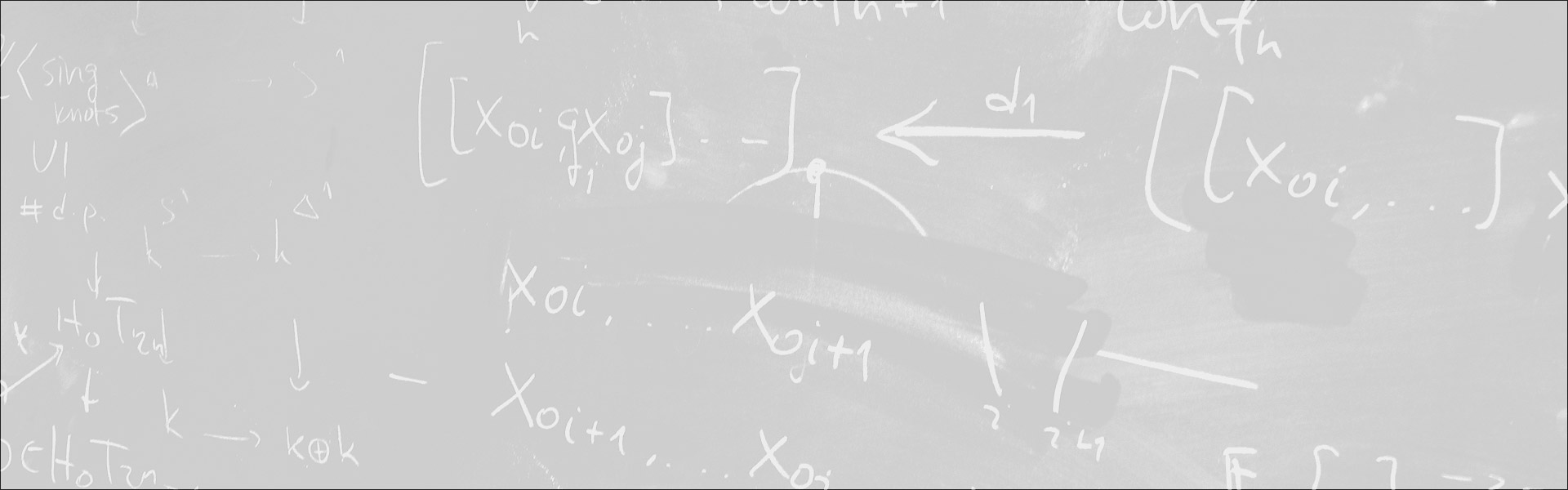Designed and built with care, filled with creative elements

Top# Nonuniqueness for the Navier–Stokes equations and model equations / Singularities in Fluid Mechanics

/  Évènements• Cet évènement est passé

## Nonuniqueness for the Navier–Stokes equations and model equations / Singularities in Fluid Mechanics

JG: In this talk, I will discuss fundamental properties of the solutions to the incompressible Navier–Stokes equations in three dimensions. After reviewing the classical local well-posedness results, I will explain how numerical simulations suggest local ill-posedness at the borderline of the known results. I will discuss a plausible scenario of non-uniqueness from smooth initial data through finite-time blow-up. Finally, I will describe how this scenario is actually happening in a model equation sharing the same fundamental properties as the Navier–Stokes equations.KM: Singularities of the Navier-Stokes equations occur when some derivative of the velocity field is infinite at any point of a field of flow (or, in an evolving flow, becomes infinite at any point within a finite time). Such singularities can be mathematical but unphysical (as e.g. in two-dimensional flow near a sharp corner) in which case they can be ‘resolved’ by improving the physical model considered; or they can be physical but non-mathematical (as e.g. in the case of cusp singularities at a fluid/fluid interface) in which case resolution of the singularity may involve incorporation of additional physical effects; these examples will be briefly reviewed. The `finite-time singularity problem’ for the Navier-Stokes equations will then be discussed and a new analytical approach will be presented; here it will be shown that there is indeed a singularity of the ‘physical but non-mathematical’ type, in that, at suf- ficiently high Reynolds number, vorticity can be amplified by an arbitrarily large factor within a finite time. In this case, the singularity is resolved by three-dimensional vortex re- connection in a manner that admits analytical description.

- Séminaire Analyse non linéaire et EDP

#### Détails :

Orateur / Oratrice : Julien Guillod et Keith Moffatt
Date : 14 mai 2019
Horaire : 9h30 - 12h00
Lieu : ENS Salle W